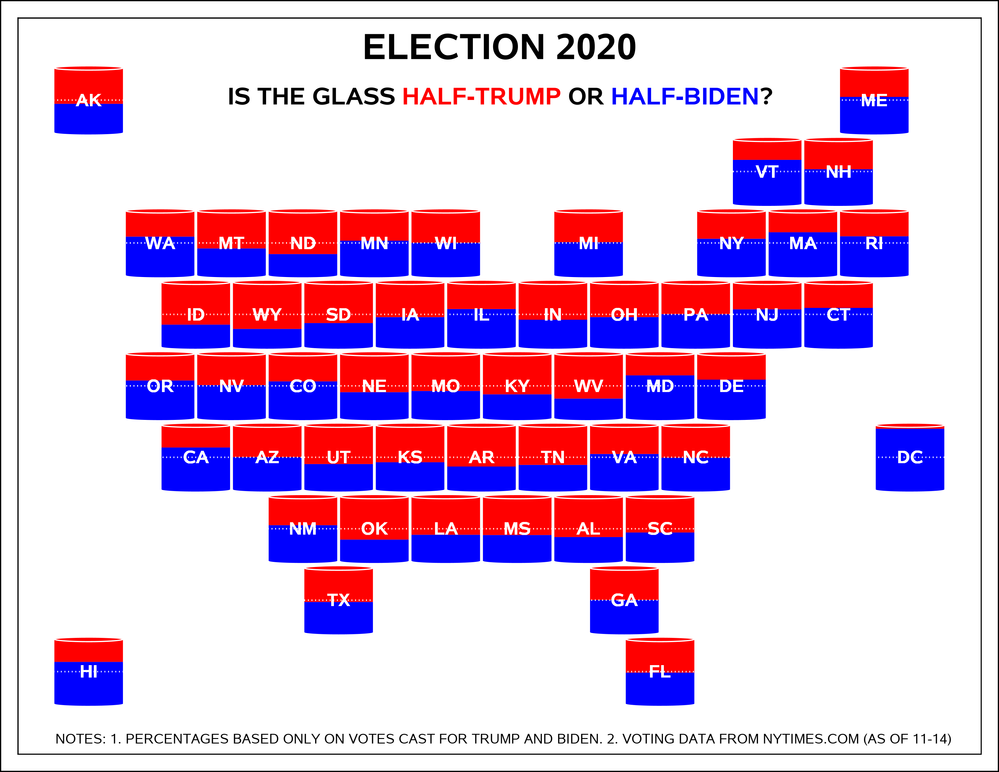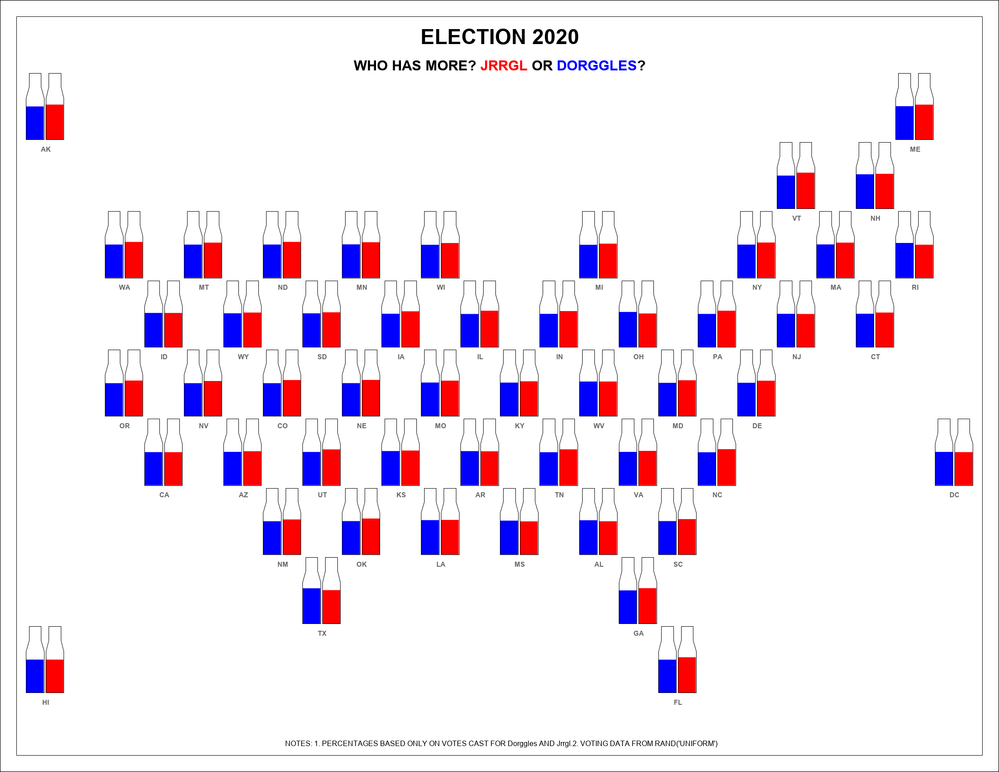## Fun With SAS ODS Graphics: Is the Glass Half-Trump or Half-Biden?A "Layered-Cocktail" Look at State Vote Totals

Yet another election map, this one a cartogram of state "glasses" showing the percentage of combined Trump and Biden votes that went to each candidate. Reference lines provided to help show when a candidate exceeds the "half-full" mark. Voting data as of 11-14 from the New York Times.

UPDATE: Modified 11-14 at 10:04 CT to fix Trump/Biden color assignment code. Output was unchanged though - managed to make two mistakes that canceled each other out. 😀

``````* Fun With SAS ODS Graphics - Is the Glass Half-Trump or Half-Biden? (Yet Another Election Map)
Data courtesy of NY Times  - nytimes.com/interactive/2020/11/03/us/elections/results-president.html;

*==> Get NY Times state-level Presidential election data (JSON format);

filename nyt '/folders/myfolders/NYTjsonResultsAsOf20201114.txt';
libname j json fileref=nyt;
* Calculate % of votes for Biden & Trump;
proc sql;                             * Dnominator is total Biden + Trump votes (others excluded);
select r.state_id,
from j.races r
left join j.races_candidates c on r.ordinal_races=c.ordinal_races
group by state_id order by r.state_id;

*==> Generate USA cartogram map x/y coordinates from inline layout of state codes;

data states(keep=statecode x y y2);
input states \$char80.;
y+1;
y2=y;                                * y-axis location of state name;
x=mod(y-1,2)*.5;                     * Offset alternate rows of states by .5;
do c=1+mod(y-1,2)*2 to 80 by 4;
statecode=substr(states,c,2);
x+1;
if statecode^='' then output;
end;
datalines;
AK                                          ME
VT, NH
WA, MT, ND, MN, WI,     MI,     NY, MA, RI
ID, WY, SD, IA, IL, IN, OH, PA, NJ, CT
OR, NV, CO, NE, MO, KY, WV, MD, DE
CA, AZ, UT, KS, AR, TN, VA, NC,         DC
NM, OK, LA, MS, AL, SC
TX              GA
HI                              FL
;
proc sort data=states; by statecode;

*==> Merge voting data and map points,
Generate points for polygons (rectangles) to make "glasses" showing each candidate's share,
Generate points for ellipses for top (Trump) and bottom (Biden) of "glasses";

by statecode;                        * Assign sort sequence for bar chart;
eyR=y-.45; output; eyR=.;            * Y-axis points for ellipses at top and bottom of "glasses";
eyD=y+.45; output; eyD=.;            * Glasses" occupy .96 of height (2*.45 for rectangles + 2*.03 for ellipses);
candidate="Biden";
p+1;                                 * Generate polygon points for Biden;
px=x-.48; py=y+.45; output; px=x+.48; output;     * Top left/right;
py=y+.45-pctBiden*.9; output; px=x-.48; output;   * Bottom left/right;
candidate="Trump";                   * Generate polygon points for Trump;
p+1;
px=x-.48; py=y-.45; output; px=x+.48; output;     * Top left/right;
py=y-.45+pctTrump*.9; output; px=x-.48; output;   * Bottom left/right;

*==> Map and bar charts of vote shares - polygon + ellipse + text plots);

ods listing image_dpi=300 gpath='/folders/myfolders';
ods graphics on / reset antialias width=11in height=8.5in imagename="PresidentialElection";

proc template;
define statgraph ustemplate;
begingraph / subpixel=on;          * Define Dem/Rep color attributes;
discreteattrmap name="candidate";
value "Trump" / fillattrs=(color=red);
value "Biden" / fillattrs=(color=blue);
enddiscreteattrmap;
discreteattrvar attrvar=candidatecolor var=candidate attrmap="candidate";
layout overlayequated / xaxisopts=(display=none thresholdmin=0 thresholdmax=0) yaxisopts=(display=none reverse=true);
layout gridded / columns=1 valign=top;  * Titles (Insets);
entry textattrs=(size=24pt weight=bold color=black) "ELECTION 2020";
entry textattrs=(size=8pt) " ";
entry textattrs=(size=16pt weight=bold color=black) "IS THE GLASS "
textattrs=(size=16pt weight=bold color=red) "HALF-TRUMP"
textattrs=(size=16pt weight=bold color=black) " OR "
textattrs=(size=16pt weight=bold color=blue) "HALF-BIDEN"
textattrs=(size=16pt weight=bold color=black) "?";
endlayout;                         * Polts to show candidates' vote shares;
polygonplot x=px y=py id=p / display=(fill) group=candidatecolor includemissinggroup=false;  * Rectangles showing candidates' vote shares;
ellipseparm semiminor=.03 semimajor=.48 xorigin=x yorigin=eyR slope=0 /
display=(fill outline) outlineattrs=(color=white pattern=solid) fillattrs=(color=red) group=eyr includemissinggroup=false;  * Top of "glass" (Trump);
ellipseparm semiminor=.03 semimajor=.48 xorigin=x yorigin=eyD slope=0 /
display=(fill) fillattrs=(color=blue) group=eyd includemissinggroup=false;  * Bottom of "glass" (Biden);
referenceline y=y / lineattrs=(pattern=dot color=white);  * Show 50%-of-vote reference lines;
textplot x=x y=y text=statecode / textattrs=(color=white weight=bold size=12pt);  * Two-digit state codes;
entry "  NOTES: 1. PERCENTAGES BASED ONLY ON VOTES CAST FOR TRUMP AND BIDEN. 2. VOTING DATA FROM NYTIMES.COM (AS OF 11-14)  " / valign=bottom;
endlayout;
endgraph;
end;
run;

proc sgrender data=statevotespolygons template=ustemplate;  * Generate the chart!;``````
6 REPLIES 6

## Re: Fun With SAS ODS Graphics: Is the Glass Half-Trump or Half-Biden?

So two wrongs makes no difference 🤔

## Re: Fun With SAS ODS Graphics: Is the Glass Half-Trump or Half-Biden?

After I clicked on the image, I still had to increase my window size to increase the map size big enough to see the 50/50 reference lines. Perhaps making them a solid line, rather than dashed, would make them show up better when the map is viewed at less than 'native' resolution?

## Re: Fun With SAS ODS Graphics: Is the Glass Half-Trump or Half-Biden?

Yea, I'm getting spoiled by higher-resolution displays. 😀 I did try solid lines, which could be seen better, but I didn't like the way they bisected the state codes (also tried backlighting letters, but felt that varied letter heights too much for this particular chart). In retrospect, I guess I probably should have tried varying the thickness of the dotted line though. Oh well, there's always next time!

## Re: Fun With SAS ODS Graphics: Is the Glass Half-Trump or Half-Biden?

Instead of a stacked bar graph inside a glass, could you do side-by-side bars inside beer bottles, and audio annotate with 100 bottles of beer on the wall.... for the bumpy bus ride we are on.

## Re: Fun With SAS ODS Graphics: Is the Glass Half-Trump or Half-Biden?

Here is the beer bottle version with side-by-side bars.```/* Modification of
* Fun With SAS ODS Graphics - Is the Glass Half-Trump or Half-Biden? (Yet Another Election Map)
* to show each opponents support in beer bottles
*
* Data randomly generated
*/

*==> Generate USA cartogram map x/y coordinates from inline layout of state codes;

data state_cartogram_points(keep=statecode x y);
length statecode \$2;
format x y 3.;
input;
line = _infile_;
if line ne: '/*';
y + 1;                                * y-axis location of state name;
do _n_ = 1 to countw(line,', ');
statecode = scan(line, _n_, ', ');
x = index(line, statecode);
output;
end;
datalines;
/* -|----10---|----20---|----30---|----40---|----50---| */
AK                                          ME
VT, NH
WA, MT, ND, MN, WI,     MI,     NY, MA, RI
ID, WY, SD, IA, IL, IN, OH, PA, NJ, CT
OR, NV, CO, NE, MO, KY, WV, MD, DE
CA, AZ, UT, KS, AR, TN, VA, NC,         DC
NM, OK, LA, MS, AL, SC
TX              GA
HI                              FL
;
proc sort; by statecode; run;

set state_cartogram_points;
by statecode;
if first.statecode;

call streaminit (123);

name = 'Jrrgl Bmurgrgly         ';
pct = round(47.5 + rand('uniform', 0, 5), 0.1);
_tot = pct;
output;

name = 'Dorggles Jrgllulrm';
do _n_ = 1 to 1000 until (_tot + pct <= 100);
pct = round(47.5 + rand('uniform', 0, 5), 0.1);
end;
_tot + pct;
output;

name = 'Erggglglia Gluggglr';
pct = 100 - _tot;
output;

keep statecode name pct;
format pct 6.2;
run;

*==> Merge voting data and map points,
Generate points for polygons (rectangles) to make "glasses" showing each candidate's share,
Generate points for ellipses for top (Trump) and bottom (Biden) of "glasses";

%macro rect(xvar=px, yvar=py, x=, y=, w=0.90, h=pct, ymult=3.5);
&xvar = &x;      &yvar = (&y + 1) * &ymult;           output;
&yvar = &yvar - &h * &ymult / 100;   output;
&xvar = &x + &w;                                      output;
&yvar = (&y + 1) * &ymult;           output;
%mend;

%macro bottles(xvar=pxo, yvar=pyo, x=x, y=y, w=0.91, h=96, ymult=3.5);
/* x1,y1 is lower left  x2,y2 is upper right */

h  = 0.96 * &ymult;
h1 = 0.60 * &ymult;
h2 = 0.76 * &ymult;

x1 = &x;         y1 = (&y + 1) * &ymult;
x2 = x1 + 0.91; y2 = y1 - h;

polygon_id+1;
&xvar = x1; &yvar = y1;  output;
&yvar = y1-h1;  output;
&xvar = x1+.15; &yvar = y1-h2;  output;
&yvar = y2; output;
&xvar = x2-.15; output;
&yvar = y1-h2; output;
&xvar = x2; &yvar = y1-h1; output;
&yvar = y1; output;

x1 + 1;
x2 + 1;
x3 + 1;
x4 + 1;

polygon_id+1;
&xvar = x1; &yvar = y1;  output;
&yvar = y1-h1;  output;
&xvar = x1+.15; &yvar = y1-h2;  output;
&yvar = y2; output;
&xvar = x2-.15; output;
&yvar = y1-h2; output;
&xvar = x2; &yvar = y1-h1; output;
&yvar = y1; output;
%mend;

options mprint;

data map_polygons (rename=name=who);
merge
state_cartogram_points
;
by statecode;

if name in: ('Jrrgl', 'Dorggles');

if name =: 'Dorggles' then dx_who = 0; else dx_who = 1;

polygon_id+1;
%rect (x=x + dx_who, y=y);
call missing (px, py);

if first.statecode then do;
pxt =  x + 1;
pyt = (y + 1) * 3.5 + .5;
output;
call missing(pxt, pyt);

%bottles ();
call missing (pxo, pyo);
end;

format px py pxt pyt pxo pyo 7.2 polygon_id dx_who 4.;
run;

options nomprint;

*==> Map and bar charts of vote shares - polygon + ellipse + text plots);

*ods listing image_dpi=300 gpath='/temp';
*ods graphics on / reset antialias width=11in height=8.5in imagename="PresidentialElection";

ods listing image_dpi=96 gpath='/temp';
ods graphics on / reset antialias width=2200px height=1700px imagename="PresidentialElection";

proc template;
define statgraph ustemplate;
begingraph / subpixel=on;          * Define Dem/Rep color attributes;

discreteattrmap name="who_colors";
value "Jrrgl Bmurgrgly"    / fillattrs=(color=red);
value "Dorggles Jrgllulrm" / fillattrs=(color=blue);
enddiscreteattrmap;

discreteattrvar
attrvar=who_color
var=who
attrmap="who_colors"
;

layout overlayequated /
xaxisopts=(display=none thresholdmin=0 thresholdmax=0)
yaxisopts=(display=none reverse=true)
;

layout gridded / columns=1 valign=top;  * Titles (Insets);
entry textattrs=(size=24pt weight=bold color=black) "ELECTION 2020";
entry textattrs=(size=8pt) " ";
entry textattrs=(size=16pt weight=bold color=black) "WHO HAS MORE? "
textattrs=(size=16pt weight=bold color=red) "JRRGL"
textattrs=(size=16pt weight=bold color=black) " OR "
textattrs=(size=16pt weight=bold color=blue) "DORGGLES"
textattrs=(size=16pt weight=bold color=black) "?";
endlayout;

polygonplot x=px y=py id=polygon_id /
display=(fill)
group=who_color
includemissinggroup=false;  * Rectangles showing candidates' vote shares;

polygonplot x=pxo y=pyo id=polygon_id /
display=(outline)
includemissinggroup=false;

textplot x=pxt y=pyt text=statecode / textattrs=(color=gray66 weight=bold size=8pt);

entry
"  NOTES: "
"1. PERCENTAGES BASED ONLY ON VOTES CAST FOR Dorggles AND Jrrgl."
"2. VOTING DATA FROM RAND('UNIFORM')  "
/ valign=bottom;
;

endlayout;
endgraph;
end;
run;

proc sgrender data=map_polygons template=ustemplate;  * Generate the chart!;
run;```

## Re: Fun With SAS ODS Graphics: Is the Glass Half-Trump or Half-Biden?

Very clever! I vote for the %bottles macro to ship with Base SAS! 😀

Discussion stats
• 6 replies
• 1067 views
• 11 likes
• 3 in conversation